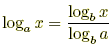index: click on a letter A B C D E F G H I J K L M N O P Q R S T U V W X Y Z A to Z index index: subject areas numbers & symbols sets, logic, proofs geometry algebra trigonometry advanced algebra & pre-calculus calculus advanced topics probability & statistics real world applications multimedia entrieswww.mathwords.com about mathwords website feedback

Logarithm Rules

Algebra rules used when working with logarithms.

 For the following, assume that x, y, a, and b are all positive. Also assume that a ≠ 1, b ≠ 1.   Definitions 1. loga x = N means that aN = x. 2. log x means log10 x. All loga rules apply for log. When a logarithm is written without a base it means common logarithm. 3. ln x means loge x, where e is about 2.718. All loga rules apply for ln. When a logarithm is written "ln" it means natural logarithm.     Note: ln x is sometimes written Ln x or LN x.   Rules 1. Inverse properties:   loga ax = x   and   a(loga x) = x 2. Product:  loga (xy) = loga x + loga y 3. Quotient:4. Power:   loga (xp) = p loga x 5. Change of base formula:Careful!! loga (x + y) ≠ loga x + loga y loga (x – y) ≠ loga x – loga y

 this page updated 19-jul-17 Mathwords: Terms and Formulas from Algebra I to Calculus written, illustrated, and webmastered by Bruce Simmons Copyright © 2000 by Bruce Simmons All rights reserved